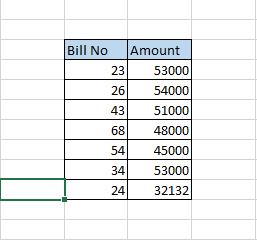Data Validation

Accounts Tax
Excel

Use of Countif Value

We know that Countif formula is of form

=COUNTIF(RANGE,CRITERIA)

This formula can also be used to find duplicate value

=countif(RAnge,SELECT ONE CELL)

If there are unique values (no duplicate) ,it will show 1

if there are 2 values,it wil show 2

if there are 3 values,it wil show 3

Example

Here,in custom field,we can put following COUNTIF Formula

COUNTIF(Select Range, Select Range in Criteria)=1

Note:-While selecting range ,we have to press F4

Q6

Prepare Data Validation on column so that no duplicate Bill No entered in columns